The Digital Abstraction

# 5. The Digital Abstraction

Having explored ways to use a binary channel capable of transferring bits between a transmitter and receiver, we now turn to the problem faced by the channel itself: physical representation of individual ones and zeros in a form that allows them to be manipulated and communicated reliably. In dealing with this problem, we will confront the deceptively complex problem of representing discrete variables as continuously-variable physical quantities; moreover, we will establish one of the major abstractions of digital systems: the combinational device.

Why digital?

## 5.1. Why digital?

In classical physics, measureable quantities that we might use to represent information -- position, voltage, frequency, force, and many others -- have values that vary continuously over some range of possibilities. The values of such variables are real numbers: even over a restricted range, e.g. the interval between 0 and 1, the number of possible values of a real variable is infinite, implying that such a variable might carry arbitrarily large amounts of information. This may appear as an advantage of continuous variables, but it comes at a serious cost: it obscures the actual information content of the variable. This limitation of the engineering discipline surrounding analog systems results in symptoms like the degraded video content of our video toolkit example of Section 4.4.

Role of Specifications

### 5.1.1. Role of Specifications

Informally, engineering involves building things: assembling components to make a system that performs some useful function. We formalize this process via specifications, both for the system and its components. Specifications abstract the external function performed by a module, and isolate that function from the internal details of the module's implementation. Ideally, a module's specifications provide all of the information an engineer using that module as a component needs to know, without encumbering that user-level view with details relevent to the implementation of the module.

Given well-designed specifications, the engineering of a system becomes the task of finding an arrangement of interconnected components that is guaranteed to meet the system specification, assuming that each of the component modules meets its module specification. Well-engineered systems are tantamount to a proof that the system "works", assuming that each of the modules work, where our standard of working is set by the specifications. While the proof is rarely formalized explicitly, it is implicit in the choices made by the system's designer.

If we are to build systems of arbitrary complexity, it is important that specifications of each module characterize that module's behavior -- viz., the information flowing into and out of each module -- precisely rather than approximately. Specifications that can only be approximated in real-world implementations, such as those for our analog video toolkit, result in systems that obey their specifications only approximately; as these systems are combined to make yet bigger systems, the approximations continue to degrade. Such models can and are used for systems of limited complexity; but to remove this complexity limit, we need an abstraction whose specifications can be simply and precisely realized in practice.

Representing discrete values: Part 1

## 5.2. Representing discrete values: Part 1

An enabling step toward the goal of simple, precisely realizable specifications is to adopt an abstract model based on discrete rather than continuous (real) variables. Although other possibilities exist, we choose the binary symbols $\{0, 1\}$ as the two distinct values that each variable may assume, so that each variable carries at most a single bit of information. The move from the unbounded information content of a continuous variable to the finite -- indeed tiny -- single bit of a binary variable represents a serious compromise in the capabilities of our model: it implies, for example, that each module's inputs and outputs each carry finite information. Paying that price, however, allows us to build devices whose specifications are obeyed precisely, and to combine the devices (and their specifications) to build systems of arbitrary complexity that obey their specifications precisely.

Given that the real-world physical variables we must use to implement our devices use continuous variables, translating our discrete-value models to real-world implementations requires a scheme for representing discrete values as real quantities like voltage. We must somehow represent 0 and 1 as distinct quantities, with no intermediate alternatives. Moreover, we must assure that the representation of a 0 is never mistaken for a 1, and vice versa. In an electronic system whose wires carry voltages between 0 and 1, we might choose that voltage less than $0.5v$ represent 0 and those above $0.5$ represent 1; however, then voltages arbitrarily close to the $0.5v$ threshold could easily be misinterpreted.Simple binary representation
We can avoid such close calls by separating the representation of 0 from that of 1 by a forbidden zone in our range of voltages, as shown in the diagram to the right. In this scheme, sufficiently low voltages (perhaps those below $0.1v$) represent a logical 0, sufficiently high voltages (perhaps those above $0.9v$) represent a 1, and those between represent neither a 0 or a 1.

While the forbidden zone allows us to reliably distinguish logical 0's from 1's, it introduces a complication: not every voltage in our systems will represent valid logic values. Indeed, given the continuous nature of our underlying physics, the voltage on a wire cannot change between representing 0 and 1 without at least temporarily going through the forbidden zone. We will address this issue shortly, via a discipline that effectivly allows us to avoid asking the question "which logic level does this voltage represent?" at time when it may be invalid.

Combinational Devices

## 5.3. Combinational Devices

Armed with a plausible approach to implementing the digital (discrete) $\{0, 1\}$ values of our model, we now introduce an abstraction for its major building block: A combinational device is a component that has
• one or more digital inputs;
• one or more digital outputs;
• a functional specification that details the logic value of each output for each combination of input values;
• a timing specification consisting, at minimum, of a propagation delay: an upper bound $t_{pd}$ on the time required for the device to compute the specified output values from an arbitrary set of stable, valid inputs.

The combinational device is our model for combinational modules, whose outputs are determined by their current inputs after a propagation delay.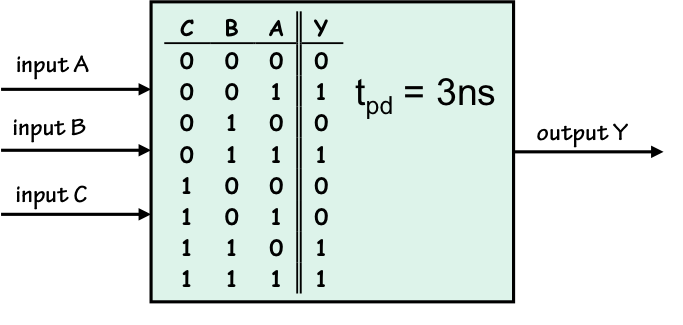Combinational Device
They are typically represented in diagrams by blocks such as that shown on the right, which shows a three-input combinational device whose functional specification is given in the form of a truth table specifying outputs for each of the $2^3$ input combinations, and whose timing specification is a 3-nanosecond upper bound on the time a valid output will appear after valid inputs are applied.
The Static Discipline

### 5.3.1. The Static Discipline

The specifications of a combinational device represent a contract between that device and its system context:
each output will be the valid logic value dictated in its specifcation whenever its inputs have been valid for at least the specified propagation delay.
Informally, combinational devices are required to produce valid outputs (after a delay) when supplied valid inputs. We call this property the static discipline, and it plays a critical role in our model: it allows us to avoid the propagation of invalid representations of logic values throughout our systems. It is static because it deals with equilibrium values at inputs and outputs (after propagation delays, which allow transients to settle); and the term discipline implies that its a self-imposed engineering constraint on the design of combinational devices.
Combinational Circuits

### 5.3.2. Combinational Circuits

A compelling property of the combinational device abstraction as a building block is the ability to build complex combinational devices by combining simpler ones. In particular, we can specify a new combinational device $S$ by a diagram of interconnected components such that
• Each component is itself a combinational device;
• Each component input is connected to either
• an input to the system $S$;
• an output of another component; or
• a constant $0$ or $1$.
• Each output of the system $S$ is connected to either
• an input to the system $S$;
• an output of a component; or
• a constant $0$ or $1$.
• The circuit contains no directed cycles, i.e, there is no cyclic path within the diagram that goes from inputs to outputs through one or more components.

Such a diagram is called a combinational circuit, and conforms to our definition of a combinational device.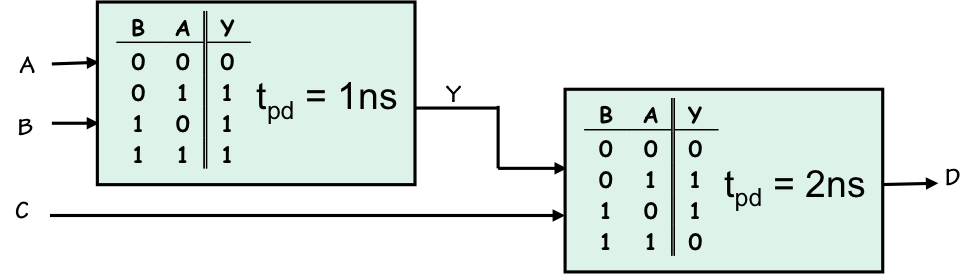A simple example is shown to the right. This diagram depicts a 3-input system using two 2-input combinational components, each having its own functional and timing specification. To see that this system is a combinational device, observe that the signal on the internal connection (labeled $Y$) is defined by the left component, and must be valid after the $A$ and $B$ inputs have been valid for 1ns (the propagation delay of the left component). Hence the inputs to the right component are specified and valid 1ns after valid $A, B, C$ inputs are applied, requiring its output to be valid after another 2ns (the propagation delay of the right component). We can thus construct a specifiation for the logical value at the circuit's output $D$ as a function of its three inputs $A, B, C$, and derive a propagation delay of 3ns for the circuit.

This analysis generalizes to combinational circuits of arbitrary size. In general we can procede through the circuit from inputs to outputs, assigning to each node in the circuit a functional specification (e.g., a truth table giving its value for each combination of circuit inputs) and a timing specification, viz. an upper bound on the time at which the signal will be valid after valid inputs have been applied to the circuit. We will see many examples of such analyses in the next chapter.

The propagation delay specification is an upper bound on the time at which a combinational device's outputs will become valid: it constitutes a performance guarantee by the device to its user. The specification may be chosen conservatively, although to extract the best performance from our systems, we tend to prefer the smallest propagation delay specifications that we can reliably guarantee. For a combinational circuit $C$, a lower bound on this value -- the tightest propagation delay we may specify -- can be computed as follows:

1. Consider each path from an input of $C$ to an output of $C$. For each such path, compute the cumulative propagation delay along that path -- i.e., the sum of the propagation delays for each component on the path.
2. Take, as the propagation delay specification for $C$, the maximum of these cumulative propagtion delays along each input-output path.
We will typically assume this bound to be the propagation delay specification of combinational circuits we design in our subsequent examples. However, as this specification is an upper bound, we may choose to specify more conservative values -- for example, to accommodate some output loading as discussed in Section 4.5.2.1.

Recall that our combinational device definition requires a timing specification that includes at minimum a single, summary propagation delay. It is sometimes useful to supply more detail in the specification; we may, for example, specify individual propagation delays for each input/output pair.

Representing discrete values: Part 2

## 5.4. Representing discrete values: Part 2

We now return to the problem of representing $0$ and $1$ logic values as voltages. Recall that we introduced a forbidden zone between ranges of voltages representing each discrete logic value, in order to reliably discriminate between representations of $0$ and $1$; this forced the distinction between valid and invalid representations on which the static discipline of our combinational device abstraction depends.

However, this new distinction -- valid/invalid representations -- confronts another difficult decision problem. We want our systems to work reliably in real-world situations in which our control over voltages on wires and their detection is imperfect: environmental noise, and manufacturing variation among devices, will perturb actual voltages from our design ideals to some limited extent.

While we have noted some real-world sources of noise in Section Section 4.5.1, we will model these imperfections here by the simple injection of a bounded amount of noise into the wires that interconnect components in our combinational circuits.In particular, we will assume that each node in our circuit is implemented as a wire whose voltage $V_{in}$ is driven by the output of some combinational device, and whose voltage $V_{out}$ is sensed by one or more inputs of other devices. We allow that the sensed voltage $V_{out}$ may differ from the applied $V_{in}$ by $V_{noise}$, an error term whose magnitude may be held with reasonable bounds by good engineering practice but not reduced to zero. The robustness of our digital systems will depend on the ability of our representation conventions to withstand some finite amount of such noise.

We might ask whether this noise-tolerant model of wires obeys the static discipline we impose on combinational devices -- i.e., does a valid representation at $V_{in}$ guarantee a valid representation at $V_{out}$?Consider the case where the applied voltage $V{in}$ is only marginally valid -- just outside the forbidden zone by a tiny fraction of a volt. The injection of a tiny $V_{noise}$ can corrupt $V_{out}$ so that it is in the forbidden zone, hence is no longer valid. If we aim to tolerate any nonzero amount of noise, we can construct an example of this sort in which a valid input is corrupted by our wire to an invalid output. Any realistic model of wires -- one that reflects any imperfections in their capacity to convey voltages between devices -- will suffer from this defect.
Noise Margins

### 5.4.1. Noise Margins

Unfortunately, we are stuck with this noise susceptibility of wires, and hence with the connections within our combinational circuits. Our recourse is to build the combinational devices themselves in such a way that they tolerate some amount of noise on the connections between them. We do this by establishing different standards of logical validity to be applied to the outputs of combinational devices that we require of their inputs: a combinational device must accept sloppy input representations but produce squeaky-clean representations at its output. The idea is that a valid signal at the output of a device will appear as a valid at a connected device input, despite a modest amount of noise introduced by the connection.

We can accomplish this goal by interspersing finite noise margins between the forbidden zone and each valid logic representation, as shown below: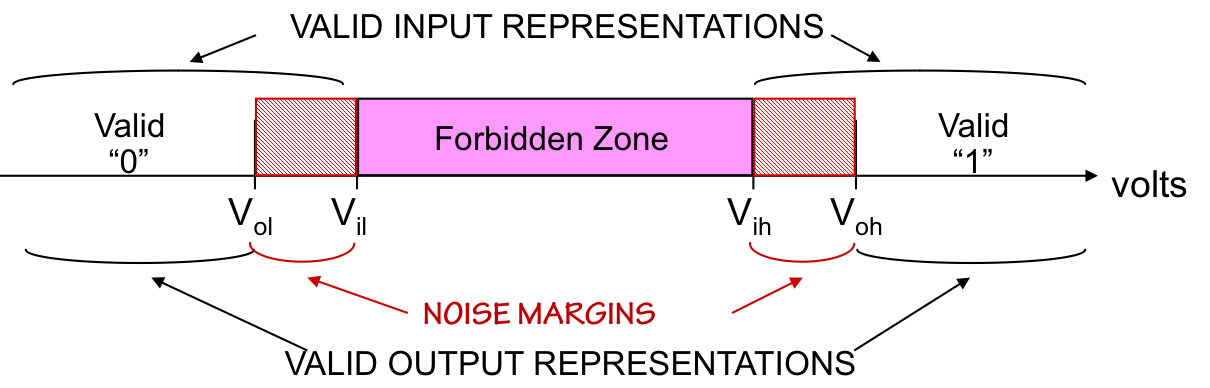The noise margins represent voltage ranges that must be accepted as valid logic levels at device inputs, but which cannot be produced as valid outputs.

We characterize our enhanced mapping between voltages and logic levels by four numeric parameters:

$V_{ol}$: The highest voltage that represents a valid $0$ at an output

$V_{il}$: The highest voltage that represents a valid $0$ at an input

$V_{ih}$: The lowest voltage that represents a valid $1$ at an input

$V_{oh}$: The lowest voltage that represents a valid $1$ at an output

The impact of the new noise margins on our combinational device abstraction is that the static discipline now requires each combinational device to accept marginally valid inputs but produce solidly valid results: the devices must restore signals that have been degraded by noise to pristine $0$s and $1$s. This is something that a wire, or any passive device, cannot do: it requires that the marginal signals be amplified by increasing their distance from the forbidden zone.
A Combinational Buffer

## 5.5. A Combinational Buffer

The need for noise margins to maintain signal validity, we have seen, rules out the use of a passive device (like a simple wire) as a combinational device that performs in our digital domain the function of the analog $Copy$ operator described in Section 4.4.Buffer
In this section, we explore constraints on the implementation of a valid combinational device that copies its digital input to its digital output, using noise margins to assure that marginal inputs are properly restored. The logic symbol for this simple building block, together with its functional specification (in the form of a truth table), is shown to the right.

When using combinational modules like the buffer as digital building blocks, we typically represent them using their logic symbol and think about their behavior in our abstracted world of $1$s and $0$s. When considering their implementation, however, we often need to pierce through the digital abstraction and consider the voltages at their input and output terminals that represent the $1$s and $0$s that are being processed.A useful tool for this exercise is a voltage transfer characteristic, a simple graph showing, for each input voltage $V_{in}$ with some range, the equilbrium output voltage $V_{out}$ if $V_{in}$ is applied and time is allowed for the $V_{out}$ to settle. Note that this curve does not involve time -- it assumes that for each $V_{in}$ we apply, $V_{out}$ will stabilize to some fixed value eventually. When we consider the voltage transfer characteristic of a combinational device, we generally assume that this settling time is covered by its propagation delay specification.

What can we say about the voltage transfer characteristic of a combinational buffer? From its functional specification, we know that a low input voltage should produce a low output voltage, and a high input voltage should produce a high voltage on its output. Given the parameters $V_{ol}$, $V_{il}$, $V_{ih}$, and $V_{oh}$ that define our logic representation, we can mark these values on both the input and output axes and reticulate our voltage input/output plane into a number of distinct regions.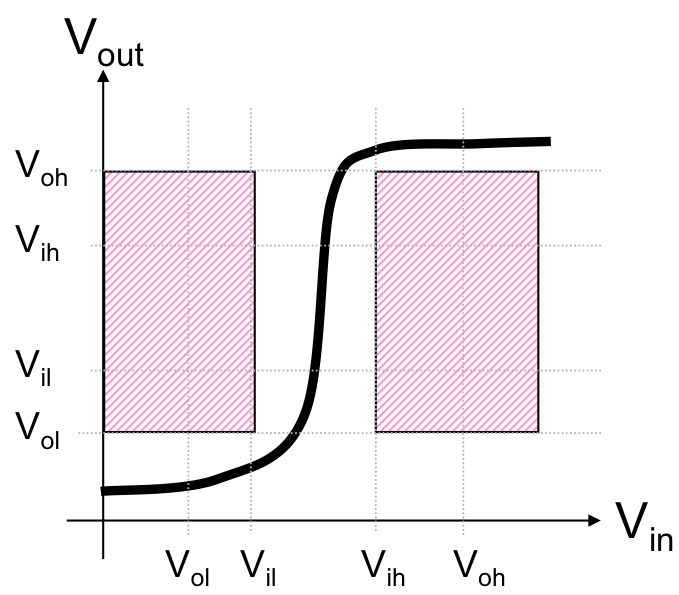Buffer VTC
Next, we can shade regions that the voltage transfer curve cannot go through if the device it represents is to obey the static discipline: those regions which represent a valid input and an invalid output. Such a shading is shown on the curve to the right. The avoidance of these regions is a simple consequence of the valid input implies valid output static discipline rule: if the transfer curve goes through such a region, then there is a valid input voltage we can apply which will yield an invalid output voltage, violating our rule.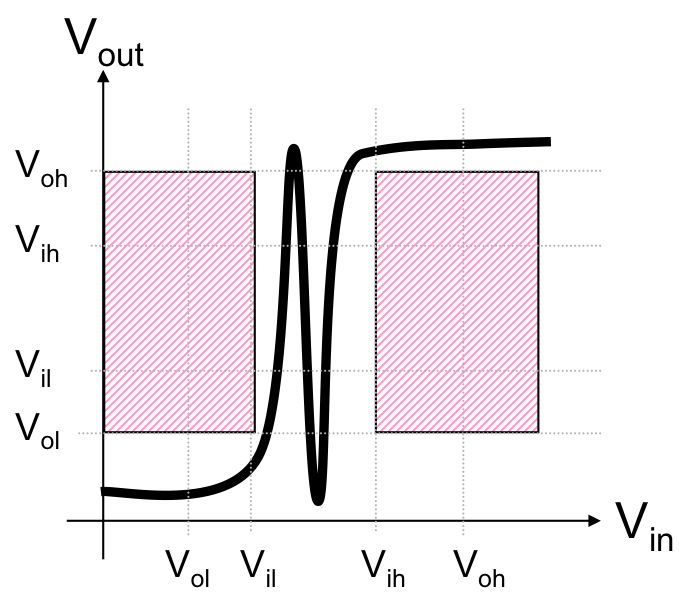The voltage transfer curve shown above dutifully avoids the outlawed shaded regions, and indeed constitutes a valid combinational buffer under the logic convention represented by the mapping parameters shown. Of course, many other curves could serve as a valid buffer, such as the somewhat wilder one shown to the left. The peculiar behavior of this curve for inputs between $V_{il}$ and $V_ih$ is confined to the forbidden zone of our logic mapping, and consequently tolerated by our combinational device abstraction.

For a combinational buffer, what we require of the voltage transfer characteristic is

1. That it obeys the functional specification (e.g., any valid 0 input yields a valid 0 output); and
2. That it avoids regions that correspond to invalid outputs and valid inputs.
It is noteworthy that the first condition implies the second; hence satisfying the functional specification (within the $t_{pd}$ time bound) establishes the validity of our combinational buffer. The first condition is specific to the logic function performed by our buffer, however, while the second applies to every single-input logic function that might be performed by a device under this logic mapping.Inverter
Suppose, for example, we wish to implement a combinational inverter -- the other "interesting" single-input logic function, whose symbol and truth table are shown to the right.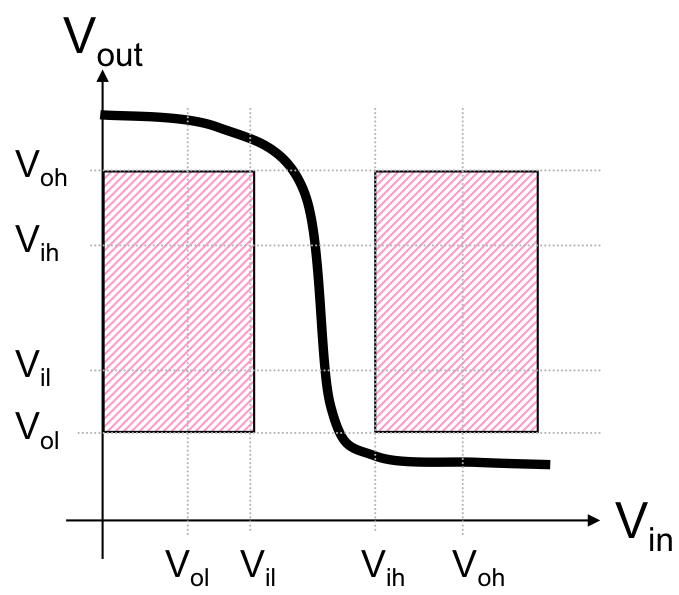Inverter VTC
Again we require that valid inputs must lead to valid outputs, so the same shaded regions of the voltage in/out plane must be avoided by the voltage transfer curve; in this case, however, the curve must go from the upper-left region ($0$ in yields $1$ out) to the lower-right ($1$ in yields $0$ out) as shown to the left.

Gain and nonlinearity requirements

## 5.6. Gain and nonlinearity requirements

The voltage transfer characteristics for each of these devices -- the buffer and inverter -- are subject to certain geometric constraints imposed by the exclusion of the shaded regions. In each case, the output actually depends upon the input; this implies that the transfer curve must go between valid $0$ and $1$ representations as the input voltage varies. This transition can only be made in the forbidden zone, between the two shaded regions of our voltage input/output plane: any other path would violate the static discipline for some range of input voltages.

Note the dimensions of the rectangular gap between the shaded regions: its width is $V_{ih}-V_{il}$, and its height is $V_{oh}-V_{ol}$. The height is necessarily greater than the width, since the height includes our noise margins $V_{il}-V_{ol}$ and $V_{oh}-V_{ih}$ that are excluded by the width. We conclude that the transfer curve must enter this tall, thin rectangle at the top (or bottom) and leave at the bottom (or top) to make the transition between $0$ and $1$. It follows that this region of the curve must be more vertical than horizontal: the magnitude of its average slope as it traverses the forbidden zone must be greater than one.

The slope of a voltage transfer curve reflects the gain of the device it describes -- the ratio between an output voltage change and the change in input voltage that caused it. The static discipline and its requirement of non-zero noise margins dictates that combinational devices exhibit gain greater than 1 (or less than -1) in the forbidden zone; this is the mechanism by which they restore marginally valid inputs to produce solid outputs. The gain requirement for combinational devices reinforces our earlier observation that a passive device (such as a wire) does not satisfy the static discipline; some active amplification is required to avoid degeneration of logic values.

It is also noteworthy that, since the input and output voltage ranges are identical, the transfer curve must "flatten out" outside of the forbidden zone to compensate for the high gain in that region. Like the example VTCs for the buffer and inverter, the slope (gain) is low for valid $0$ and $1$ inputs, but high between them. The curve must be nonlinear, since its slope necessarily varies over the range of voltages that may be applied. This, along with the requirement for amplification (gain), rules out the use of a simple linear device like a resistor as a combinational device.

Constraints on logic mapping parameters

## 5.7. Constraints on logic mapping parameters

Digital designers don't ordinarily get to choose the logic mapping parameters $V_{ol}$, $V_{il}$, $V_{ih}$, and $V_{oh}$; they are specifications dictated by the family of logic devices being used. Since our goal is a single logic representation scheme to be used by a wide range of devices and operating circumstances, the parameters for a logic family tend to be chosen conservatively. The choice is heavily dependent on the underlying technology being used, as well as other factors such as anticipated range of environmental conditions (temperature, electrical noise, etc) and variability of the manufacturing process.

For reliable operation, it is desireable to maximize the noise margins. If we model noise as the injection of a bounded perturbation $V_{noise}$ in connections between devices, we may view noise immunity, defined as the lesser of the two noise margins, as a limit to the magnitude of $V_{noise}$ that our family will tolerate. For this reason, we prefer to equalize the noise margins to the extent possible.

The choice of the output parameters $V_{ol}$ and $V_{oh}$ is restricted to the voltages that can be reliably produced by the technology of the logic family. Given values for the output parameters, the choice of valid input ranges dictated by $V_{il}$ and $V_{ih}$ is subject to two opposing goals: (a) the desire to maximize noise margins, and (b) the need for every device in the family to obey the static discipline (i.e., for its transfer curve to avoid forbidden regions).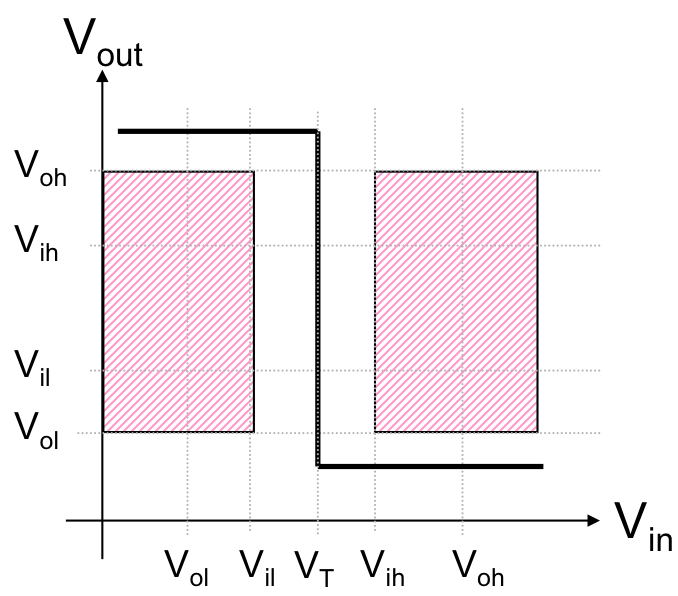Ideal inverter VTC
These goals are best satisfied by a technology whose voltage transfer curve offers very high gain at some tightly controlled voltage threshold $V_T$, such as that shown on the right. In this ideal case, the device offers (negative) infinite gain at $V_{in}=V_T$, and zero gain at other input voltages. Given this characteristic, we could expand the noise margins by moving $V_{il}$ and $V_{ih}$ closer to the $V_T$ threshold voltage, expanding our shaded regions until they almost touch the transfer curve. The extent to which we can do this is limited, however, by the variability of the manufacturing process and operating conditions. If $V_T$ differs from device to device due to uncontrollable factors (as it will), or is temperature dependent (as it is likely to be), our conservative selection of $V_{il}$ and $V_{ih}$ must allow for these variations.
Combinational Timing

## 5.8. Combinational Timing

Our combinational device abstraction provides a simple guarantee on the timing of output signal validity: whenever all inputs to a combinational device have been stable and valid for the specified propagation delay for that device, each output is guaranteed to be a valid representation of the value dictated by the functional specification for the device. Beyond this promise, there are no validity guarantees on the outputs; in particular, unless all inputs have been valid for at least a propagation delay, an output may be a valid $0$, a valid $1$, or invalid.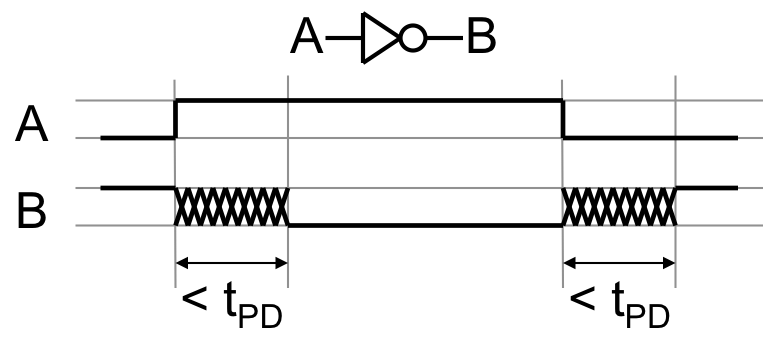Inverter timing
It is convenient to visualize the timing relationships between signals via a timing diagram such as that shown to the right. The diagram shows the timing of an inverter whose input $A$ is an "ideal" voltage waveform having two instantaneous transitions; each transition causes the inverter output $B$ to assume a new valid logic value after the prescribed propagation delay $t_{PD}$. Note that our timing diagram abstracts away details of the output signal behavior during the propagation delay: the crosshatched regions of the output waveform indicate, symbolically, that the output voltage is unspecified during these intervals. It may assume the valid output value earlier than required; it may oscillate between valid $0$ and $1$ during this period; or it may assume an invalid level in the forbidden zone. In the real-world circuits we build, we will typically see an exponential decay to the valid output value, but our abstraction leaves this detail completely unspecified.

As the diagram indicates, the duration of each unspecified interval is bounded by $t_{PD}$; keep in mind that this propagation delay is a device specification, and applies to all instances of the inverter and all operating conditions anticipated in its design. It is chosen to be conservative, providing a guaranteed upper bound to the settling time of the output.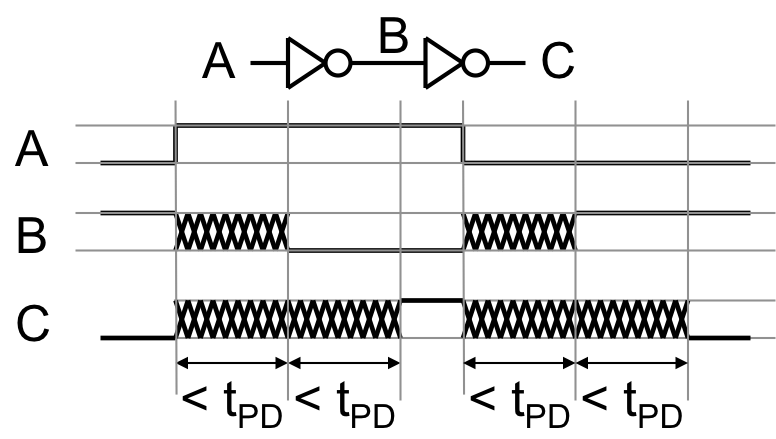The instantaneous transitions on the input waveform mask another important contractual detail in our combinational timing model: the output guarantee applies a propagation delay after all inputs become valid. If the input transition takes finite time (which, realistically, is always the case) we must allow time for a valid logic value to be reached before starting the $t_{PD}$ clock. To illustrate, consider adding a second inverter to our little test circuit, resulting in the timing diagram shown above. The output of the second inverter is unspecified for a $t_{PD}$ while its input $B$ -- the output of the first inverter -- is unspecified, and for a $t_{PD}$ following that interval.
Optional stronger guarantees

## 5.9. Optional stronger guarantees

While the combinational device abstraction as described thus far serves the majority of our needs well, there are occasional circumstances where stronger guarantees are necessary. We will encounter an important such case in Chapter 8, when we use combinational devices to build digital memory elements.

In this section, we introduce two extensions to the combinational device abstraction to deal with such extenuating circumstances. Each amounts to an additial guarantee that can be specified for a combinational device, extending its timing or functional specification, respectively, beyond our usual standard.

Contamination Delay

### 5.9.1. Contamination Delay

Our combinational device abstraction provides for a propagation delay specification, explicitly recognising the physical reality that it takes some finite time for information in the inputs of a device to propagate to its outputs. The propagation delay is an upper bound on a period of output invalidity; this specification is conservatively chosen, since invalidity of logic signals can cause systems to fail.

One can argue for recognition of another, symmetric physical reality: given a device with valid inputs and outputs, it takes finite time for the fact that an input has become invalid to propagate to an output and contaminate its validity. Our model makes the pessimistic assumption that any invalid input immediately contaminates all outputs: all output guarantees are instantly voided by any invalid input. This pessimism is, again, conservative: systems can fail due to invalidity of logic levels, not due to undocumented validity for a brief period after an input change.

It is occasionally useful, however, to rely on an output remaining valid for a brief interval after an input becomes invalid. To support these infrequent needs, we introduce a new, optional specification that can augment a combinational device:

contamination delay $t_{CD}$: a specified lower bound on the time a valid output will persist following an input becoming invalid.

In the usual case, we assume that the contamination delay of a combinational device is zero -- that is, that an invalid input immediately contaminates (renders invalid) all outputs. In cases where we require a nonzero contamination delay, it reflects a minumum time it will take an invalid input to propagate to (and contaminate) an output.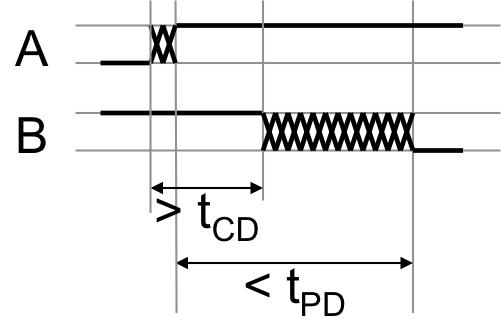Inverter Contamination Delay
The figure to the right shows the timing constraints on a combinational inverter having a nonzero contamination delay specification. Note that the previously valid output is required to remain valid for $t_{CD}$ following input invalidity, and is still required to become valid (with its new output value) $t_{PD}$ following the establishment of newly valid inputs. It is important to note that the $t_{CD}$ interval begins at the time the input becomes invalid, while $t_{PD}$ starts when the input becomes valid.

In Section 5.3.2, we noted that an acyclic circuit whose components are combinational devices is itself a combinational device, and observed that an appropriate specification for the propagation delay of the circuit is the maximum cumulative propagation delay over every input-to-output path through the circuit. This maximum corresponds to our understanding of propagation delay as an upper bound on the time for valid input values to propagate to each output.

In contrast, we can specify a contamination delay for the circuit as the minimum cumulative contamination delay over every input-to-output path through the circuit; this stems from our definition of contamination delay as a lower bound on the time for an invalid input value to propagate to any output.

We will see many examples of combinational circuits and their timing specifications in Chapter 7.

Lenient combinational devices

### 5.9.2. Lenient combinational devices

Combinational devices are not required to produce valid outputs unless each of their inputs are valid; using the terminology of denotational semantics, they compute strict functions of their inputs. This characteristic of combinational devices works fine for most of our logic needs, and in the vast majority of cases, all inputs to a combinational device will be valid for a propagation delay before we depend on the validity of its output.

However, it is possible (and occasionally useful) to make stronger guarantees, particularly in situations where the output of a function can be deduced when input values have been only partially specified.Combinational
OR
Consider, for example, a 2-input OR gate - a combinational device computing the function shown in the truth table to the right. As there are two combinational inputs, there are $2^2=4$ input combinations hence 4 rows to the truth table. If we tie the $A$ input to $1$ and vary the $B$ input between $0$ and $1$, the combinational device specification allows the output $Y$ to be unspecified (or invalid) for a propagation delay after each input change. Since we're holding $A$ to $1$, however, we see that the truth table requires $Y=1$ for either $B=0$ or $B=1$ -- in this case, the output is independent of the input $B$.

It is possible to build combinational devices that exploit situations in which the output is determined by a subset of the input values, and guarantee valid outputs in such cases. We call such devices lenient (or non-strict) combinational devices, and define them as follows:

A lenient combinational device is a combinational device whose output is guaranteed valid whenever any combination of inputs sufficient to determine the output value has been valid for at least $t_{PD}$.

Notice that a lenient combinational device is, in fact, a combinational device; it is one offering an additional guarantee that it will produce a valid output when only a subset of its inputs are valid, to the extent possible.Lenient OR
We often rewrite the truth tables of lenient devices having variables or don't care values in the input columns, as shown to the left. Here the * in an input column can be read as "any value, valid or not"; the "* 1" line, for example, explicitly guarantees that so long as $B=1$ the output $Y$ will be $1$.

Lenience is a convenient property, and is occasionally essential; we will see such a case in Chapter 8. Happily, basic gates made using our technology of choice as described in Chapter 6 are lenient, although more complex circuits are often not lenient unless special care is taken in their design. We will revisit this topic in Chapter 7.

Chapter Summary

## 5.10. Chapter Summary

In this chapter, we have taken a first major step toward the engineering abstraction that underlies digital system engineering: the representation of the discrete variables of our logic model using underlying continuously variable physical parameters. Our representation conventions take care to allow manufacturable devices to conform to realizable specifications made in the logic domain: it is robust in the presence of noise, parasitics, and manufacturing variations that inevitably plague real-world systems.

Key elements of this engineering discipline include:

• Our 4-parameter mapping convention, using $V_{ol}$, $V_{il}$, $V_{ih}$, and $V_{oh}$ to partition a continuous range of voltages into five distinct regions which define valid representations of logical $1$s and $0$s;
• The separation of valid $0$ and $1$ representations by voltage ranges which do not represent $1$ or $0$;
• The combinational device, an abstract model of logic elements whose specifications include a functional specification (e.g., a truth table) and a propagation delay $t_{pd}$, and which guarantees valid outputs whenever all inputs have been valid for at least $t{pd}$;
• The incorporation of noise margins in our logic mapping, requiring the outputs of combinational devices to adhere to stricter validity standards than the inputs they accept. This important property forces each combinational device to restore marginally valid logic signals to unequivocally valid $1$s and $0$s;
• The fact that acyclic circuits whose components are combinational devices are, themselves, combinational devices. This gives us a simple construction rule for building large systems from a repertoire of small building blocks;
• An optional contamination delay specification, which provides an optional guarantee that outputs of a combinational device remain valid for a short interval after inputs become invalid; and
• The optional stronger guarantee offered by a lenient combinational device, which guarantees valid outputs in cases where functionally irrelevent inputs are invalid.
In many cases contamination delay and lenience are unimportant, and combinational devices can be assumed to be non-lenient and have zero contamination delay. We will see shortly, however, situations in which these optional guarantees play a critical role in our model.

The combination of these ingredients results in a simple engineering model that largely allows the designer of a system to ignore the complexities of voltages, phase, noise, and other real-world complications, working entirely in the abstracted world of $1$s, $0$s, and truth tables.